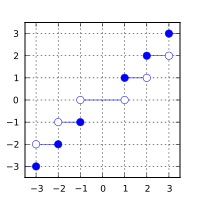/C++

# std::trunc, std::truncf, std::truncl

Defined in header `<cmath>`
(1)
```float       trunc ( float num );
double      trunc ( double num );
long double trunc ( long double num );```
(until C++23)
```constexpr /* floating-point-type */
trunc ( /* floating-point-type */ num );```
(since C++23)
`float       truncf( float num );`
(2) (since C++11)
(constexpr since C++23)
`long double truncl( long double num );`
(3) (since C++11)
(constexpr since C++23)
Defined in header `<cmath>`
```template< class Integer >
double      trunc ( Integer num );```
(A) (constexpr since C++23)
1-3) Computes the nearest integer not greater in magnitude than `num`. The library provides overloads of `std::trunc` for all cv-unqualified floating-point types as the type of the parameter. (since C++23)
 `double` (since C++11)

### Parameters

 num - floating-point or integer value

### Return value

If no errors occur, the nearest integer value not greater in magnitude than `num` (in other words, `num` rounded towards zero) is returned.

Return value`num`

### Error handling

Errors are reported as specified in `math_errhandling`.

If the implementation supports IEEE floating-point arithmetic (IEC 60559),

• The current rounding mode has no effect.
• If `num` is ±∞, it is returned, unmodified
• If `num` is ±0, it is returned, unmodified
• If `num` is NaN, NaN is returned

`FE_INEXACT` may be (but isn't required to be) raised when truncating a non-integer finite value.

The largest representable floating-point values are exact integers in all standard floating-point formats, so this function never overflows on its own; however the result may overflow any integer type (including `std::intmax_t`), when stored in an integer variable.

The implicit conversion from floating-point to integral types also rounds towards zero, but is limited to the values that can be represented by the target type.

The additional overloads are not required to be provided exactly as (A). They only need to be sufficient to ensure that for their argument `num` of integer type, `std::trunc(num)` has the same effect as `std::trunc(static_cast<double>(num))`.

### Example

```#include <cmath>
#include <initializer_list>
#include <iostream>

int main()
{
const auto data = std::initializer_list<double>
{
+2.7, -2.9, +0.7, -0.9, +0.0, 0.0, -INFINITY, +INFINITY, -NAN, +NAN
};

std::cout << std::showpos;
for (double const x : data)
std::cout << "trunc(" << x << ") == " << std::trunc(x) << '\n';
}```

Possible output:

```trunc(+2.7) == +2
trunc(-2.9) == -2
trunc(+0.7) == +0
trunc(-0.9) == -0
trunc(+0) == +0
trunc(+0) == +0
trunc(-inf) == -inf
trunc(+inf) == +inf
trunc(-nan) == -nan
trunc(+nan) == +nan```

 floorfloorffloorl (C++11)(C++11) nearest integer not greater than the given value (function) ceilceilfceill (C++11)(C++11) nearest integer not less than the given value (function) roundroundfroundllroundlroundflroundlllroundllroundfllroundl (C++11)(C++11)(C++11)(C++11)(C++11)(C++11)(C++11)(C++11)(C++11) nearest integer, rounding away from zero in halfway cases (function) C documentation for `trunc`